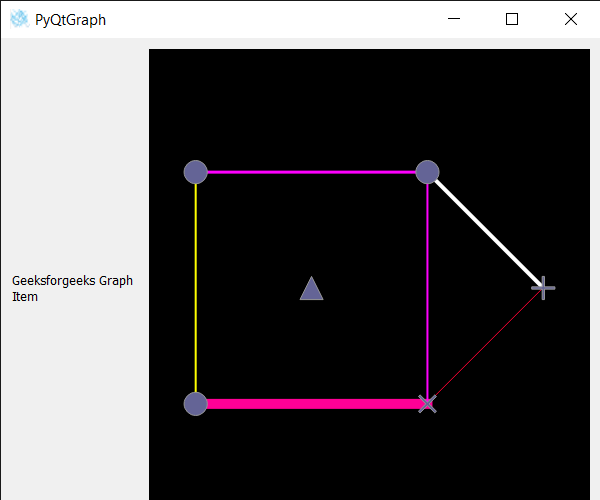# PyQtGraph – Setting Pen of Graph Item

• Last Updated : 08 Dec, 2021

In this article we will see how we can set the pen of the graph item in PyQTGraph. PyQtGraph is a graphics and user interface library for Python that provides functionality commonly required in designing and science applications. Its primary goals are to provide fast, interactive graphics for displaying data (plots, video, etc.). A Graph is a non-linear data structure consisting of nodes and edges. The nodes are sometimes also referred to as vertices and the edges are lines or arcs that connect any two nodes in the graph. Graph consists of a finite set of vertices(or nodes) and set of Edges which connect a pair of nodes. Pen is used to draw the line of graph i.e joining edges, by setting pen we can set the color, size and opacity of each line.

We can create a graphic layout widget and graphic item with the help of command given below

```# creating graphics layout widget
win = pg.GraphicsLayoutWidget()

# creating a graph item
graph_item = pg.GraphItem()```

In order to do this we use setPenmethod with the graph item object
Syntax : imv.setPen(lines)
Argument : It takes numpy array as argument
Return : It returns None

Below is the implementation

## Python3

 `# importing Qt widgets``from` `PyQt5.QtWidgets ``import` `*` `# importing system``import` `sys` `# importing numpy as np``import` `numpy as np` `# importing pyqtgraph as pg``import` `pyqtgraph as pg``from` `PyQt5.QtGui ``import` `*``from` `PyQt5.QtCore ``import` `*``import` `pyqtgraph.ptime as ptime`  `# Image View class``class` `ImageView(pg.ImageView):` `    ``# constructor which inherit original``    ``# ImageView``    ``def` `__init__(``self``, ``*``args, ``*``*``kwargs):``        ``pg.ImageView.__init__(``self``, ``*``args, ``*``*``kwargs)` `class` `Window(QMainWindow):` `    ``def` `__init__(``self``):``        ``super``().__init__()` `        ``# setting title``        ``self``.setWindowTitle(``"PyQtGraph"``)` `        ``# setting geometry``        ``self``.setGeometry(``100``, ``100``, ``600``, ``500``)`  `        ``# icon``        ``icon ``=` `QIcon(``"skin.png"``)` `        ``# setting icon to the window``        ``self``.setWindowIcon(icon)` `        ``# calling method``        ``self``.UiComponents()` `        ``# showing all the widgets``        ``self``.show()` `    ``# method for components``    ``def` `UiComponents(``self``):` `        ``# creating a widget object``        ``widget ``=` `QWidget()` `        ``# creating a label``        ``label ``=` `QLabel(``"Geeksforgeeks Graph Item"``)` `        ``# setting minimum width``        ``label.setMinimumWidth(``130``)` `        ``# making label do word wrap``        ``label.setWordWrap(``True``)` `        ``# setting configuration options``        ``pg.setConfigOptions(antialias``=``True``)` `        ``# creating graphics layout widget``        ``win ``=` `pg.GraphicsLayoutWidget()` `        ``# adding view box to the graphic layout widget``        ``view ``=` `win.addViewBox()` `        ``# lock the aspect ratio``        ``view.setAspectLocked()` `        ``# creating a graph item``        ``graph_item ``=` `pg.GraphItem()` `        ``# adding graph item to the view box``        ``view.addItem(graph_item)` `        ``# Define positions of nodes``        ``pos ``=` `np.array([``            ``[``0``, ``0``],``            ``[``10``, ``0``],``            ``[``0``, ``10``],``            ``[``10``, ``10``],``            ``[``5``, ``5``],``            ``[``15``, ``5``]``        ``])` `        ``# Define the set of connections in the graph``        ``adj ``=` `np.array([``            ``[``0``, ``1``],``            ``[``1``, ``3``],``            ``[``3``, ``2``],``            ``[``2``, ``0``],``            ``[``1``, ``5``],``            ``[``3``, ``5``],``        ``])` `        ``# Define the symbol to use for each node (this is optional)``        ``symbols ``=` `[``'o'``, ``'x'``, ``'o'``, ``'o'``, ``'t'``, ``'+'``]` `        ``# setting data to the graph item``        ``graph_item.setData(pos``=``pos, adj``=``adj, size``=``1``, symbol``=``symbols, pxMode``=``False``)` `        ``# Creating a grid layout``        ``layout ``=` `QGridLayout()` `        ``# minimum width value of the label``        ``label.setMinimumWidth(``130``)` `        ``# setting this layout to the widget``        ``widget.setLayout(layout)` `        ``# adding label in the layout``        ``layout.addWidget(label, ``1``, ``0``)` `        ``# plot window goes on right side, spanning 3 rows``        ``layout.addWidget(win, ``0``, ``1``, ``3``, ``1``)` `        ``# setting this widget as central widget of the main window``        ``self``.setCentralWidget(widget)`  `        ``# Define the line style for each connection (this is optional)``        ``lines_pen ``=` `np.array([``            ``(``255``, ``0``, ``150``, ``255``, ``10``),``            ``(``255``, ``0``, ``255``, ``255``, ``2``),``            ``(``255``, ``0``, ``255``, ``255``, ``3``),``            ``(``255``, ``255``, ``0``, ``255``, ``2``),``            ``(``255``, ``0``, ``50``, ``255``, ``1``),``            ``(``255``, ``255``, ``255``, ``255``, ``4``),``        ``], dtype``=``[(``'red'``, np.ubyte), (``'green'``, np.ubyte), (``'blue'``, np.ubyte), (``'alpha'``, np.ubyte), (``'width'``, ``float``)])` `        ``# setting pen to the graph item``        ``graph_item.setPen(lines_pen)` `# create pyqt5 app``App ``=` `QApplication(sys.argv)` `# create the instance of our Window``window ``=` `Window()` `# start the app``sys.exit(App.``exec``())`

Output :My Personal Notes arrow_drop_up